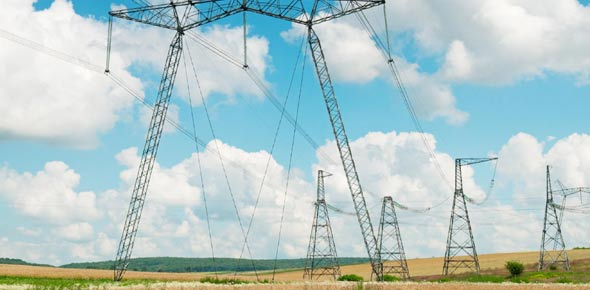# Additional Electric Current And Resistance

10 Questions | Attempts: 1337SettingsElectric current is flow of electricity through objects. As you are studying to become an electrical engineer one of the basic things to ensure you understand is how electric current and resistance. Take up the quiz below and see if you are ready to dive deeper into your course work. All the best as you tackle it!

• 1.
In which direction does electric current flow around a circuit.
• A.

Clockwise

• B.

Anti-clockwise

• C.

Positive to Negative

• D.

Negative to Positive

• E.

Left to right

• 2.
When an electric current flows through a wire what is moving?
• A.

Molecules move along the wire

• B.

Atoms move along the wire

• C.

Protons move along the wire

• D.

Electrons move along along the wire

• E.

Ions move along the wire

• 3.
A current of 2.5A flows through a light bulb with a potential difference of 10V applied across it. What is the resistance of the light bulb?
• A.

0.25 ohms

• B.

4 ohms

• C.

7.5 ohms

• D.

12.5 ohms

• E.

25 ohms

• 4.
A 60 ohm heater is used in a car heating system. If a potential difference of 12V is applied, what is the current that flows through the heater.
• A.

0.2A

• B.

5A

• C.

48A

• D.

72A

• E.

720A

• 5.
Which of the following best describes the behaviour of a Thermistor.
• A.

Its resistance increases if the voltage increases

• B.

Its resistance decreases if the voltage increases

• C.

Its resistance decreases if the current increases

• D.

Its resistance increases if the temperature increases

• E.

Its resistance decreases if the temperature increases

• 6.
An electrical device that will only let the electric current flow in one direction is called
• A.

A Bulb

• B.

A Battery

• C.

An LDR

• D.

A Diode

• E.

A Termistor

• 7.
Two resistors are connected in series. If the resistors are 20 ohms and 30 ohms the total resistance of the circuit is
• A.

0.67 ohms

• B.

1.5 ohms

• C.

10 ohms

• D.

50 ohms

• E.

600 ohms

• 8.
A 10 ohm resistor and a 50 ohm resistor are connected in parallel. The best description of the combined resistance is
• A.

Less than 10 ohms

• B.

10 ohms

• C.

Between 10 ohms and 50 ohms

• D.

50 ohms

• E.

More than 50 ohms

• 9.
In a parallel circuit the component with the largest resistance gets:-
• A.

The most potential difference

• B.

The least potential difference

• C.

The most current

• D.

The least current

• E.

All components get the same current

• 10.
As the current through a filament light bulb, its resistance increases. The best reason for this is:-
• A.

The light gets brighter

• B.

The filament gets hotter

• C.

The potential difference increases

• D.

The current increases

• E.

The filament vibrates

## Related TopicsBack to top
×

Wait!
Here's an interesting quiz for you.